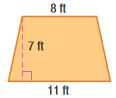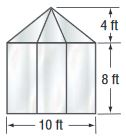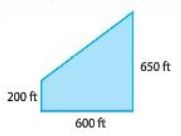Homework Explained - Math Practice 101Dear guest, you are not a registered member. As a guest, you only have read-only access to our books, tests and other practice materials.

As a registered member you can:

Registration is free and doesn't require any type of payment information. Click here to Register.
Go to page:
Chapter 9: Mid-Chapter Review

### Problem Solving

• Question 1

Find the height of a deck if it is a parallelogram with base $$8\frac{1}{4}$$ feet and an area of $$49\frac{1}{2}$$ square feet.

•  h = feet
• Question 2

How much material is needed to make a triangular flag with base $$2\frac{1}{4}$$ feet and height $$8\frac{1}{2}$$ feet?

• A =
• square feet
• Question 3

One room in Franco’s room is in the shape of a trapezoid with the dimensions shown. Find the area of the wall to be painted.• A=
• square feet
• Question 4

Reason Abstractly A window is shaped like a trapezoid. The bases are 30 inches and 40 inches. The height of the window is 24 inches. Find the area of the window.

•  square inches
• Question 5

A bricklayer wants to arrange 16 bricks into a rectangular shape with the greatest perimeter possible. How many bricks will be in each row?

•  Greatest perimeter: units
• Question 6

Identify Structure Each side of a rectangle with a length of 10 centimeters, and a width of 8 centimeters is multiplied by 0.5. Describe the change in the perimeter.

•  The new perimeter is: the size of the original perimeter
• Question 7

The side of a four season room is being constructed with the dimensions shown. Find the area of the side being built of glass windows.•  A = square feet

### Family Land

The Hemandez family owns a plot of land as shown.• Question 8

Part A

A house on the land measures 45 feet by 38 feet, a wooded area covers 118 feet by 60 feet, and the front yard is 78 feet by 40 feet.The remainder of the land is farmed. How many acres of the land are farmed? Round to the nearest tenth. Explain your answer.(Hint: 1 acre = 43,560 square feet)

• Question 8

Part B

It costs $0.05 per square foot to seed crops on 4 acres. The remainder of the farm land is seeded with grass for grazing animals. It costs$0.03 a square foot to seed the grass. What is the total cost to seed the farm land?